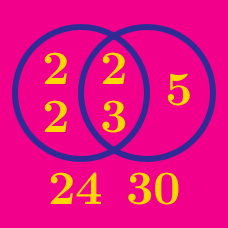Number Theory

# Greatest Common Divisor / Lowest Common Multiple: Level 5 Challenges

I've posted a question, so to celebrate, I decided to play pool. Somehow my house has tables with holes at four corners of any dimensions. The ball and the holes are negligibly small (epsilon by epsilon).

My mathematical friend says, "I will give you a $m\times n$ rectangular table where $m, n$ are positive integers summing to 2015. It's up to me to decide. Hahaha. Then we will play." Then he will put the ball at one corner.

I am very bad at aiming, so I only have a machine that will shoot the ball at an angle of 45° to the sides of the table. My evil friend wants the ball to bounce as many times as possible before it reaches any hole so that the ball can lose as much kinetic energy as possible and leave me in suspense.

I calculate that the maximum number of bounces is $M$.

For how many ordered pairs $(m, n)$ does the number of bounces equal $M$?

Clarifications:

As an explicit example, a $1\times2$ table needs 1 bounce, and a $2\times3$ table needs 3 bounces. A $1\times2014$ table needs 2013 bounces.

You may make the assumption that we are decent mathematicians, although we can't play pool and do weird things. Note also that this is combinatorics, not computer science.

$\large \sum_{n=1}^\infty \dfrac{\gcd(n,2016)}{n^2}= \dfrac{a}{b}\pi^2$

If the equation above holds true for positive integers $a$ and $b$, find $a+b$.

Clarification:
$\gcd(m,n)$ denotes the greatest common divisor of $m$ and $n$.

A positive integer $n$ is called ingenious if $\displaystyle\sum_{m=1}^{n} \gcd (m,n)$ is a prime. Find the number of ingenious integers between $3$ and $100$ (inclusive).

Details and assumptions

• You may refer to this list of primes.

• As an explicit example, when $n=3,$ we have $\displaystyle\sum_{m=1}^{3} \gcd (m, 3) = \gcd (1, 3) + \gcd (2, 3) + \gcd (3, 3)= 5,$ which is a prime.

• This problem was inspired by IMOSL 2004 N2.

$\Large A =2017^{40^{640}} - 2017 \qquad \qquad B = 2017^{40^{544}} - 2017$

The greatest common divisor of $A$ and $B$ can be expressed in the form $\large 2017^{x^{y}} - 2017$, where $x$ and $y$ are integers.

Submit your answer as $\overline{xy}$, which is the concatenation of the digits of $x$ and $y$. For example, if $x = 10$ and $y = 12$, then $\overline{xy} = 1012$.

Find the largest integer $n$ such that $n$ is divisible by all positive integers less than $\sqrt{n}$.

×